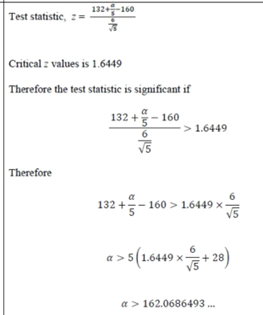# Central Limit Theorem: How do you calculate the test statistic?Paper: 6691/01R Edexcel GCE Statistics S3 June 2014R

Hello! I'm wondering why the test statistic's denominator is 6/(square root of 5). The central limit theorem says population variance is equal to the sample variance, but why do we sometimes have the denominator of the square root of (population variance and sample variance)?

Question 4: A manufacturing company produces solar panels. The output of each solar panel is normally distributed with a standard deviation of 6 watts. It is thought that the mean output is 160 watts.
A researcher believes that the mean output of the solar panels is greater than 160 watts. He writes down the output values of 5 randomly selected solar panels. He uses the data to carry out a hypothesis test at the 5% level of significance. He tests H0: mu= 160 against H1: mu > 160
On reporting to his manager, the researcher can only find 4 of the output values. These are shown below:
168.2 157.4 173.3 161.1
Given that the result of the hypothesis test is that there is significant evidence to reject H0 at the 5% level of significance, calculate the minimum possible missing output value.Original post by helloyoongles
Paper: 6691/01R Edexcel GCE Statistics S3 June 2014R

Hello! I'm wondering why the test statistic's denominator is 6/(square root of 5). The central limit theorem says population variance is equal to the sample variance, but why do we sometimes have the denominator of the square root of (population variance and sample variance)?

Question 4: A manufacturing company produces solar panels. The output of each solar panel is normally distributed with a standard deviation of 6 watts. It is thought that the mean output is 160 watts.
A researcher believes that the mean output of the solar panels is greater than 160 watts. He writes down the output values of 5 randomly selected solar panels. He uses the data to carry out a hypothesis test at the 5% level of significance. He tests H0: mu= 160 against H1: mu > 160
On reporting to his manager, the researcher can only find 4 of the output values. These are shown below:
168.2 157.4 173.3 161.1
Given that the result of the hypothesis test is that there is significant evidence to reject H0 at the 5% level of significance, calculate the minimum possible missing output value.

It's not really using the central limit theorem. It's not about comparing sample and population distributions.

When you estimate the mean of the distribution, the reliability increases when you collect more data. The variance of the mean estimate is sigma^2/n, where sigma^2 is the variance of the original distribution. The random variable here is the mean estimate.
(edited 2 years ago)Original post by mqb2766
It's nothing to do with the central limit theorem. It's not about comparing sample and population distributions.

When you estimate the mean of the distribution, the reliability increases when you collect more data. The variance of the mean estimate is sigma^2/n, where sigma^2 is the variance of the original distribution. The random variable here is the mean estimate.

Ooo thank you so much!! I understand it now.Hi,
For the same question, i dont understand how they got X in the test statistic (132+alpha/5)Original post by acha.n
Hi,
For the same question, i dont understand how they got X in the test statistic (132+alpha/5)

Using the usual definition of z, it should correspond to the sample mean?but where did they get 132+alpha/5 fromOriginal post by acha.n
but where did they get 132+alpha/5 from

Youre given 4 values and the 5th is the variable alpha. So the sample mean is ...ohhh so the sample mean is (660 + alpha)/5 which simplifies to 132+ alpha/5. Thats great! but where did they get 1.6449 from, i know it's z.
(edited 3 weeks ago)Original post by acha.n
ohhh so the sample mean is (660 + alpha)/5 which simplifies to 132+ alpha/5. Thats great! but where did they get 1.6449 from, i know it's z.

Its a one sided test at 5% level so ...
It would help (you) to post your working as its just following the usual definitions.(edited 3 weeks ago)Im not sure if this is useful, but i still dont underneayhOriginal post by acha.n
Im not sure if this is useful, but i still dont underneayh

Cant see the image, though it could be due to the "improvements" on the forum.

Just type in a few lines with a short description. The OP didnt have the 1.6449 in so Im assuming youre looking at a mark scheme or something?Yes the markscheme:I just dont get why its more than 1.6449, as i put less than 0.05 since that is the significance level to reject the original hypothesis.Original post by acha.n
I just dont get why its more than 1.6449, as i put less than 0.05 since that is the significance level to reject the original hypothesis.

Without being funny, try not to look at the mark schemes too much and sketch a bell distribution (pdf) and mark the relevant info on.

5% or 0.05 is the area of the right tail. The important X value is the critical value at the start of the corresponding critical region.

the normal pdf has a mean of 160 and std dev of 6/sqrt(5)

Youre testing whether the sample mean of 132+alpha/5 lies in, or outside the critical region.

1.64 represents how many standard deviations (6/sqrt(5)) is the critical value greater than the mean (160). Multiplying 6/sqrt(5) by 1.64 and adding it to 160, gives the critical value.

A sketch is arguably the key thing, rather than reading values from the mark scheme.
(edited 3 weeks ago)